QPalette

## Detailed Description¶

The PySide.QtGui.QGradient class is used in combination with PySide.QtGui.QBrush to specify gradient fills.

Qt currently supports three types of gradient fills:

• Linear gradients interpolate colors between start and end points.
• Simple radial gradients interpolate colors between a focal point and end points on a circle surrounding it.
• Extended radial gradients interpolate colors between a center and a focal circle.
• Conical gradients interpolate colors around a center point.

A gradient’s type can be retrieved using the PySide.QtGui.QGradient.type() function. Each of the types is represented by a subclass of PySide.QtGui.QGradient :

 PySide.QtGui.QLinearGradient PySide.QtGui.QRadialGradient PySide.QtGui.QConicalGradient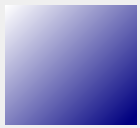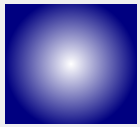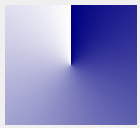The colors in a gradient are defined using stop points of the QGradientStop type; i.e., a position and a color. Use the PySide.QtGui.QGradient.setColorAt() function to define a single stop point. Alternatively, use the PySide.QtGui.QGradient.setStops() function to define several stop points in one go. Note that the latter function replaces the current set of stop points.

It is the gradient’s complete set of stop points (accessible through the PySide.QtGui.QGradient.stops() function) that describes how the gradient area should be filled. If no stop points have been specified, a gradient of black at 0 to white at 1 is used.

A diagonal linear gradient from black at (100, 100) to white at (200, 200) could be specified like this:

```linearGrad = QLinearGradient(QPointF(100, 100), QPointF(200, 200))
```

A gradient can have an arbitrary number of stop points. The following would create a radial gradient starting with red in the center, blue and then green on the edges:

```radialGrad = QRadialGradient(QPointF(100, 100), 100)
```

It is possible to repeat or reflect the gradient outside its area by specifiying the spread method using the PySide.QtGui.QGradient.setSpread() function. The default is to pad the outside area with the color at the closest stop point. The currently set spread method can be retrieved using the PySide.QtGui.QGradient.spread() function. The QGradient.Spread enum defines three different methods: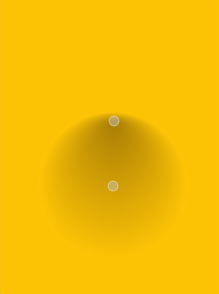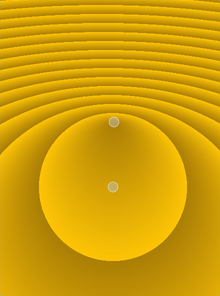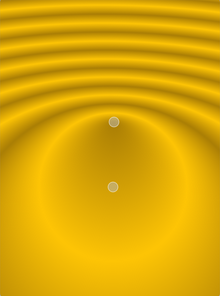PadSpread RepeatSpread ReflectSpread

Note that the PySide.QtGui.QGradient.setSpread() function only has effect for linear and radial gradients. The reason is that the conical gradient is closed by definition, i.e. the conical gradient fills the entire circle from 0 - 360 degrees, while the boundary of a radial or a linear gradient can be specified through its radius or final stop points, respectively.

The gradient coordinates can be specified in logical coordinates, relative to device coordinates, or relative to object bounding box coordinates. The coordinate mode can be set using the PySide.QtGui.QGradient.setCoordinateMode() function. The default is LogicalMode , where the gradient coordinates are specified in the same way as the object coordinates. To retrieve the currently set coordinate mode use PySide.QtGui.QGradient.coordinateMode() .

This enum specifies how gradient coordinates map to the paint device on which the gradient is used.

Constant Description
QGradient.LogicalMode This is the default mode. The gradient coordinates are specified logical space just like the object coordinates.
QGradient.StretchToDeviceMode In this mode the gradient coordinates are relative to the bounding rectangle of the paint device, with (0,0) in the top left corner, and (1,1) in the bottom right corner of the paint device.
QGradient.ObjectBoundingMode In this mode the gradient coordinates are relative to the bounding rectangle of the object being drawn, with (0,0) in the top left corner, and (1,1) in the bottom right corner of the object’s bounding rectangle.

Specifies how the area outside the gradient area should be filled.

Constant Description

Constant Description
Constant Description
QGradient.ComponentInterpolation The color components and the alpha component are independently linearly interpolated.
QGradient.ColorInterpolation The colors are linearly interpolated in premultiplied color space.

Returns the coordinate mode of this gradient. The default mode is LogicalMode .

Returns the interpolation mode of this gradient. The default mode is ColorInterpolation .

Returns true if the gradient is the same as the other gradient specified; otherwise returns false.

Returns true if the gradient is the same as the other gradient specified; otherwise returns false.

Parameters: pos – PySide.QtCore.qreal color – PySide.QtGui.QColor

Creates a stop point at the given position with the given color . The given position must be in the range 0 to 1.

Sets the coordinate mode of this gradient to mode . The default mode is LogicalMode .

Sets the interpolation mode of this gradient to mode . The default mode is ColorInterpolation .

Note that this function only has effect for linear and radial gradients.

Parameters: stops –